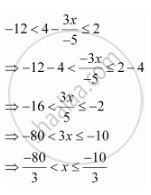# Solve the Inequality -12 < 4 - (3x)/(-5) <= 2 - Mathematics

Solve the inequality  -12 < 4 - (3x)/(-5) <= 2

#### SolutionThus, the solution set for the given inequality is (-80/3 , -10/3)

Concept: Algebraic Solutions of Linear Inequalities in One Variable and Their Graphical Representation
Is there an error in this question or solution?
Chapter 6: Linear Inequalities - Miscellaneous Exercise [Page 132]

#### APPEARS IN

NCERT Class 11 Mathematics
Chapter 6 Linear Inequalities
Miscellaneous Exercise | Q 5 | Page 132
Share